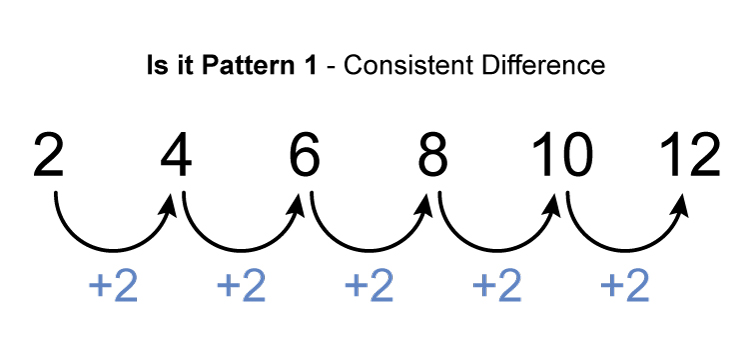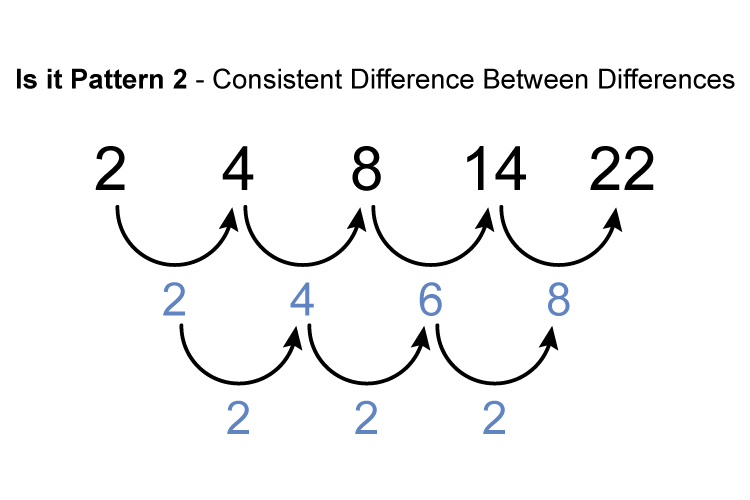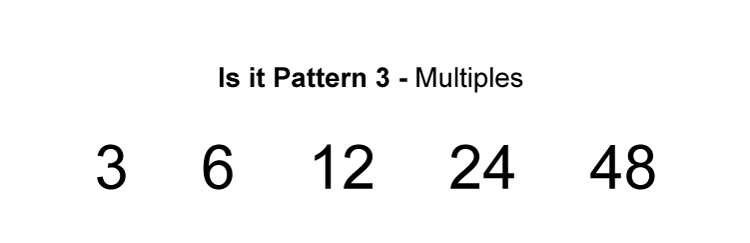# Sequence and patterns order of easiness

To identify a pattern in a sequence you should first see if you recognise a sequence straight away. If not you should tackle this in the following order of easiness.There is a consistent difference of 2 between each number.There is a consistent difference between the difference of the sequence and that number is 2.Divide each term by the previous term.

6/3=2     12/6=2    24/12=2    48/24=2